# Graphs and Graph Algorithms¶

The use of graphs is widespread in computing. Many everyday and practical problems can be modelled on graphs. We'll start out by looking at how to construct and draw a few kinds of graphs and then discuss some fundamental algorithms we can use with them. To actually carry out the computations, we'll be using a Python module called NetworkX.

Recall that a graph consists of a collection of nodes and a collection of edges between them. Graphs typically come in two flavors: undirected and directed, each having their own purpose. Here are a few model problems where each type of graph may occur.

Our first problem deals with an internet service provider who would like to expand its services and provide fiber optic internet to a town. Of course, they want to do this at the lowest cost.

In order to figure out the best way to do this, we'll model the locations we must connect as nodes in a graph and potential lines of cable to run as (undirected) edges. In addition, these edges are weighted by a cost due to things such as the cost of cable or the labor that goes into digging trenches for the cable. How should we solve this problem?

Of course, this is a rather simplified version of a more realistic problem, as we're focused on a narrow sense of connectivity. A more reasonable requirement would build in some redundency. For example, if a cable were damaged, the entire network wouldn't go down.

Our second problem deals with scheduling. What we've got is a bunch a tasks which must be completed; the catch is that some of the tasks can't be started until other ones are complete. For example, if you are building a house, you must excavate the ground before laying the foundation. On the other hand, determining the kind of lights or wallpaper you would like is completely independent and someone else can do this simultaneously.

We'll model this problem as a directed graph where the tasks are the nodes and the directed edges encode dependencies between tasks. So, maybe there is an edge "Task A -> Task B" if "Task B" requires "Task A" to be completed first. We'll see that there is a nice algorithm called topological sorting which gives us an ordered list of tasks which ensures that all dependencies are met as we complete the list.

## Creating and Drawing Graphs¶

We will be using the library networkx for most of our graph related work and start with creating various types of graphs and drawing them.

NetworkX is NOT meant for fancy graph drawing although with some effort it can be made to.

Graph drawing is hard and is best left to specialized programs listed in NetworkX drawing documentation. However NetworkX does provide some basic drawing capability through Matplotlib which they say may be removed in future versions. We will be using this functionality for now.

### Some special graphs¶

Let us start with creating and drawing some of the graphs that appear in the beginning of the book Graph Theory by Frank Harary. The first two graphs to appear in the book are in the dedication to Kuratowski who proved a seminal theorem about graph planarity. These two graphs, the complete graph $K_5$ with 5 vertices and the complete bipartite graph $K_{3,3}$ play a leading role in that theorem.

Create and draw $K_5$:

In :
%matplotlib inline

In :
import networkx as nx
import matplotlib.pyplot as plt

K5 = nx.complete_graph(5)
nx.draw(K5)
plt.show()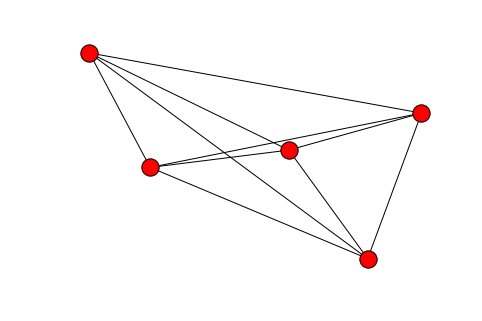The above figure does not look like the classic pentagonal shape that $K_5$ is typically drawn as. The drawings are made by networkx using matplotlib. So some things are controlled by networkx and some by manipulating matplotlib. For example, the circular layout produces a pentagonal shape:

In :
nx.draw_circular(K5)
plt.show()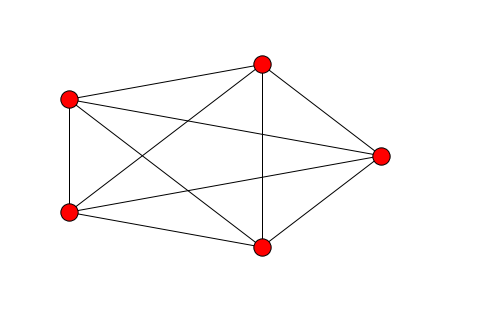That's better, but perhaps you were expecting an upright regular pentagon. You can make it regular quite easily by making sure that the axis is draw with equal scale for $x$ and $y$ directions. Since the plotting is being done by matplotlib you can do this, for example, as follows:

In :
nx.draw_circular(K5)
ax = plt.gca()
ax.set_aspect('equal')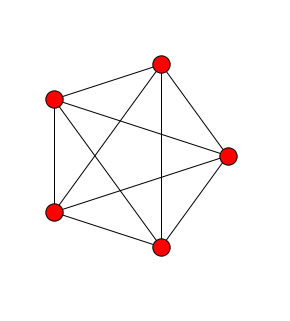To make it upright, you would have to specify the coordinates for the nodes and use the draw() or draw_networkx() function. Computing the nodes is possible but we will leave the plot as is. Perhaps there is also a way to rotate the figure by $\pi/2$ counterclockwise, but if so then that is left to the reader.

Let us now repeat the above exercise with $K_{3,3}$.

In :
K33 = nx.complete_bipartite_graph(3,3)
nx.draw(K33)
plt.show()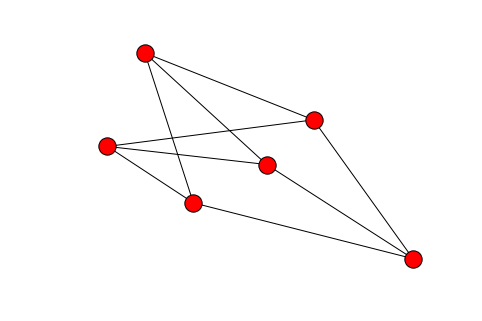This looks terrible. You can hardly recognize the familiar shape of $K_{3,3}$ at first glance. Lets redraw this with more control. For this we need to specify the locations of the nodes. We don't know which nodes form which part of the bipartite graph. And we can't even see the node numbers above.

The function networkx.draw_networkx gives more control on the drawing and also draws the node numbers by default.

In :
nx.draw_networkx(K33)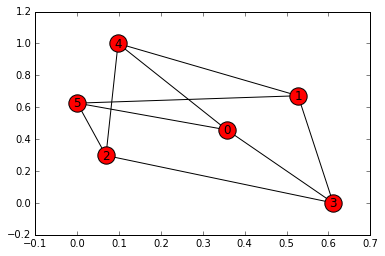Now a glance at the above figure shows that 3, 4, 5 form one part and the remaining form the other part. We can see this because there are no edges amongst 3, 4, 5. So in the next figure we will place 0,1,2 on one horizontal row and 3,4,5 below them in another horizontal row.

In :
positions = {0:[-1,1], 1:[0,1], 2:[1,1], 3:[-1,-1], 4:[0,-1], 5:[1,-1]}
nx.draw_networkx(K33, positions)
plt.show()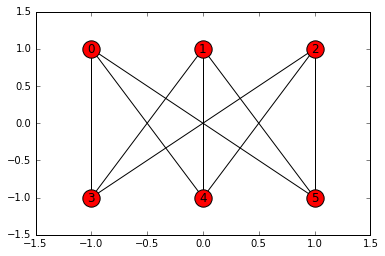This looks like the more familiar picture of $K_{3,3}$. Note that the matplotlib axis is drawn by default when using draw_networkx(). To get rid of it we have to get a handle to the axis and turn off the drawing of axis and pass that axis to draw_networkx(). The following code achieves this.

In :
ax = plt.figure().gca()
ax.set_axis_off()
nx.draw_networkx(K33, positions)
plt.show()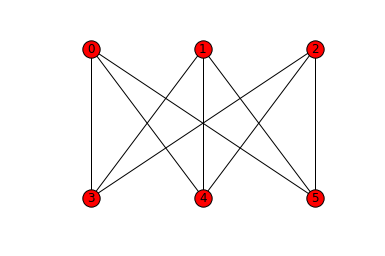You may not want to see the nodes as colored, or may want to change the color, or may want a different shape for the nodes. You may or may not want to label the nodes. All of this can be accomplished from the keyword arguments of draw_networkx(). Lets draw $K_5$ and $K_{3,3}$ in the classic graph theory textbook style with nodes drawn as solid filled small circles without numbering.

In :
ax = plt.figure().gca()
ax.set_axis_off()
options = {'node_size' : 100, 'node_color' : 'k'}
nx.draw_networkx(K33, positions, with_labels=False, **options)
plt.show()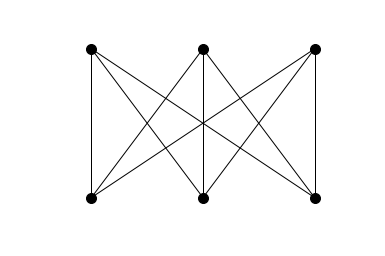Networkx has functions for creating other special graphs. Some examples of such graphs with arbitrary number of nodes are: balanced tree, cycle, grid, hypercube, path, wheel, star and others. You can find details in the Networkx documentation in the Graph Generators section.

### Hamilton's around the world puzzle¶

Another graph that is mentioned in the introductory chapter in Harary's book is the graph that appears in a puzzle Sir William Hamilton created in 1859. In this puzzle the 20 vertices of a regular dodecahedron are labelled by city names. You have to create a tour of these cities that visits each city exactly once. This game can be played on the graph formed by the vertices and edges of the regular dodecahedron. A picture of this graph from Harary's book is shown below.There is a function in Networkx to create this graph. Let's create and draw the dodecahedral graph.

In :
H = nx.dodecahedral_graph()
nx.draw_networkx(H)
plt.gca().set_aspect('equal')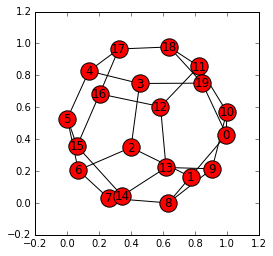The default drawing in this case is not planar like the figure from Harary's book. We can approximate that figure by using a shell layout for drawing. In this kind of a layout the vertices are laid out in concentric circles. Our attempt will get the shells correct but not the ordering or positioning of the vertices.

In :
front_face = [0, 10, 11, 18, 19]
back_face = [5, 6, 7, 14, 15]
middle = list(set(range(20)).difference(front_face + back_face))
shells = [front_face] + [middle] + [back_face]
positions = nx.shell_layout(H, shells)
nx.draw_networkx(H, positions)
ax = plt.gca()
ax.set_aspect('equal')
ax.set_axis_off()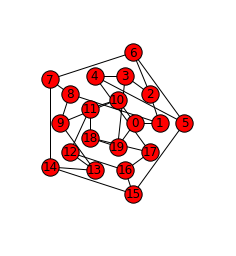To get a layout like Harary's we would have to reorder or rearrange the nodes in one or more shells as given to shell_layout(). It may be easier to just fix the positions of all vertices by hand rather than using shell_layout(). But we will leave this as an exercise and move on.

### Creating graphs the hard way¶

Graphs can also be built from scratch edge by edge and node by node. Here is one example of how that might be done.

In :
G = nx.Graph()

plt.figure()
nx.draw(G, with_labels=True)
plt.show()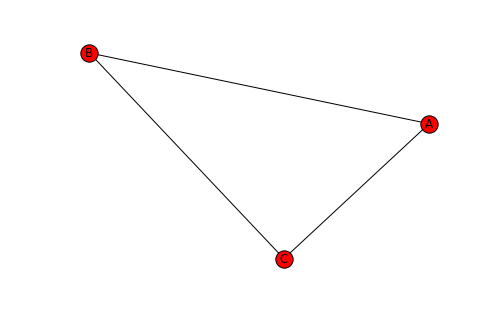This can be done with fewer commands by giving lists of nodes and edges.

In :
G = nx.Graph()

G.add_edges_from([('A', 'B'), ('B', 'C'), ('C', 'A')])

plt.figure()
nx.draw(G, with_labels=True)
plt.show()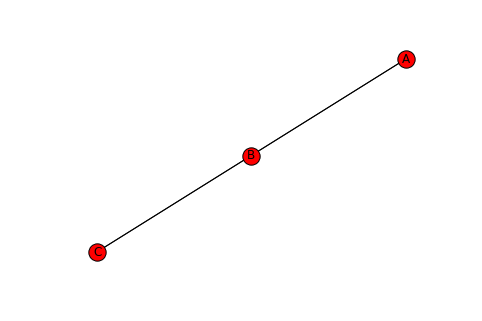### Directed graphs¶

All the examples we have seen above are undirected in that the direction of the edges doesn't matter. If the direction is important we can use DiGraph object which gives us instances of directed graphs.

Lets recreate the previous graph as a directed graph.

In :
G = nx.DiGraph()

G.add_edges_from([('A', 'B'), ('B', 'C'), ('C', 'A')])

plt.figure()
nx.draw(G, with_labels=True)
plt.show()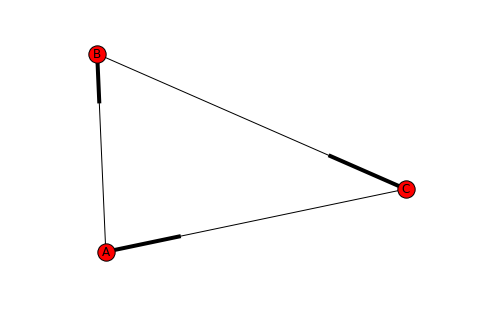The edges are drawn differently and indicate the direction. The following single edge graphs highlight this difference.

In :
G = nx.DiGraph()

plt.figure()
nx.draw(G, with_labels=True)
plt.show()

G = nx.DiGraph()

plt.figure()
nx.draw(G, with_labels=True)
plt.show()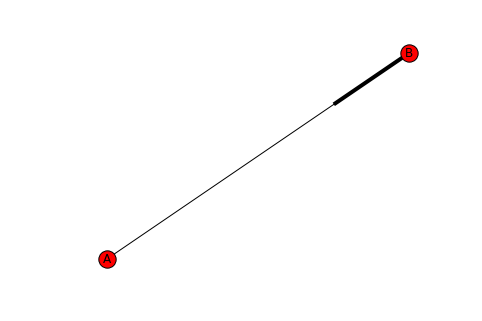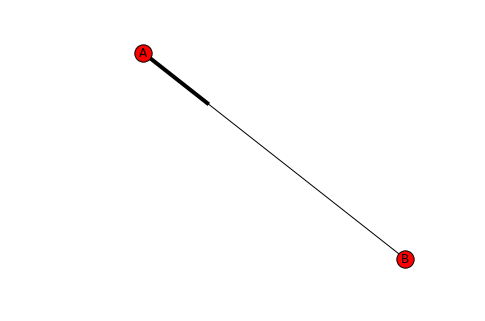### Multigraphs and the seven bridges of Königsberg¶

Now lets continue with some more examples from Harary's book. Another example in the book is the multigraph of the Königsberg bridge problem. This was the problem that led to the creation of the field of graph theory in the hands of Euler. Through the city of Königsberg flowed the river Pregel. (The city is now known as Kaliningrad and is in Russia. The river is known as Pregloya.) There are 2 large islands that are part of the city. These were connected to each other and the mainland by 7 bridges. You can see a recent sattelite view on Google maps. The number and locations of the bridges are now different from Euler's times.

Let's divide the mainland into the north shore and the south shore and call the two islands the west island (which is the smaller one) and east island. The west island was connected to the north shore by 2 bridges and to the south shore by 2 bridges. The east island was connected to the north and south shores by 1 bridge each. The two islands were connected to each other by 1 bridge. Thus there were a total of 7 bridges.

The problem that Euler solved was: is there a walk starting and ending at the same point that takes you over each bridge exactly once. We will represent the land masses by nodes that we will label north, south, east and west. The bridges will be edges. This makes the graph naturally a multigraph -- a graph in which there can be multiple edges between nodes and self-loops (edges from a node to itself). In the present case we don't have self-loops. But there are 2 edges between west and north etc. The following code makes the Königsberg multigraph.

In :
import networkx as nx

K = nx.MultiGraph()
('west', 'south'), ('west', 'south')])


Let's explore the multigraph just made.

In :
K.degree()

Out:
{'east': 3, 'north': 3, 'south': 3, 'west': 5}
In :
K.edges()

Out:
[('west', 'east'),
('west', 'north'),
('west', 'north'),
('west', 'south'),
('west', 'south'),
('east', 'north'),
('east', 'south')]
In :
K.neighbors('west')

Out:
['east', 'north', 'south']
In :
K.neighbors('south')

Out:
['west', 'east']

Unfortunately drawing the multigraph in Networkx is not satisfying, because multiple edges are drawn as single edges and self-loops are not drawn.

As mentioned earlier, there is a way to draw multigraphs properly using an interface from Networkx to Graphviz, which is a well-developed piece of software. There is a Python package called pygraphviz to access Graphviz. It is easy to install. But we will not use it here and move on to the next example.

### Kirchhoff's electrical networks¶

As mentioned on page 3 of Harary's book, another early use of graphs was in the work of Kirchhoff who studied electrical networks. He showed how to solve electrical network problems using spanning trees. The following figure shows an example of an electrical network containing 1 capacitor, 4 resistors and 3 inductors.Kirchhoff's technique would replace the above network by the underlying graph, without distinguishing the types of elements. The corresponding graph is drawn by the following code. The above example contains a single element between each pair of node, so we will just use a Graph object. In general an electrical network can have elements in parallel and so a MultiGraph would be more suitable in general. Let us label the 3 nodes in the top row as 0, 1, 2 and the nodes on the bottom row as 3, 4, 5.

In :
import networkx as nx
import matplotlib.pyplot as plt

N = nx.Graph()
resistors = [(0,1), (1,2), (3,4), (4,5)]
capacitors = [(0,2)]
inductors = [(0,3), (1,4), (2,5)]


For clarity we separated the list of edges corresponding to each type of electrical element. Note that we did not create the nodes before adding the edges. The nodes that don't exist when an edge is added are created automatically. Let us check the nodes and edges in the graph just created.

In :
N.nodes()

Out:
[0, 1, 2, 3, 4, 5]
In :
N.edges()

Out:
[(0, 1), (0, 2), (0, 3), (1, 2), (1, 4), (2, 5), (3, 4), (4, 5)]

When we draw the graph just created we will notice another unfortunate aspect of the Nteworkx built-in drawing function draw_networkx().

In :
positions = {0:(-1,0.5), 1:(0,0.5), 2:(1,0.5), 3:(-1,0), 4:(0,0), 5:(1,0)}
ax = plt.figure().gca()
ax.set_axis_off(); ax.set_aspect('equal')
options = {'node_size':50, 'node_color':'k', 'with_labels':False}
nx.draw_networkx(N, pos=positions, ax=ax, **options)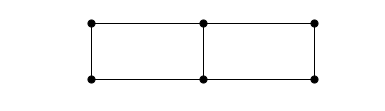Notice that the capacitor edge from the top left to top right was not draw. This is because of edges are drawn as straight line and so that edge overlaps with the edges of the 2 top horizontal edges. To see all the edges let's draw the graph again using random positions for the node.

In :
ax = plt.figure().gca()
ax.set_axis_off()
options = {'node_size':50, 'node_color':'k', 'with_labels':False}
nx.draw_networkx(N, ax=ax, **options)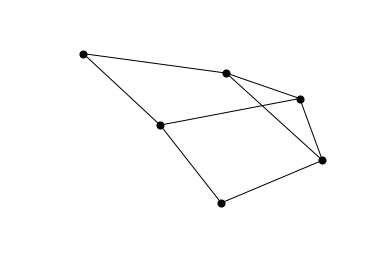Now we see all 8 edges. For variety lets draw this again with the draw() function and with the node numbering turned on but node color matching the white background.

In :
nx.draw(N, node_color=(1,1,1))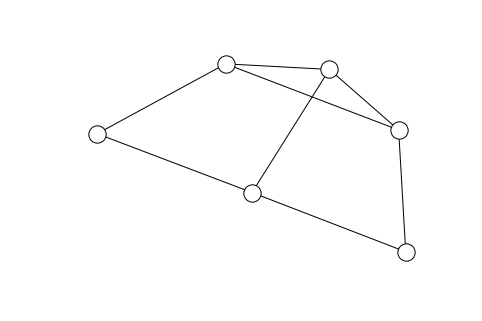Note that the positioning is different each time we draw with random positions for the nodes. Lets see this with the draw() function again.

In :
nx.draw(N, node_color=(1,1,1))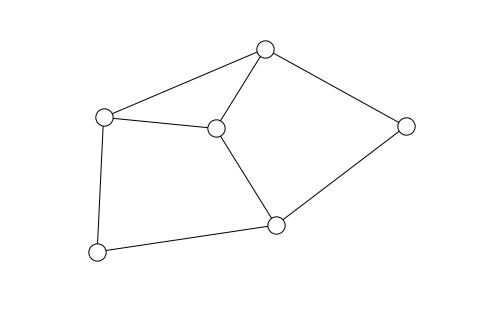There are many other functions for creating graphs with variable number of nodes. For example one can generate graphs of the following (and many other) types: balanced tree, cycle, grid, hypercube, path, wheel, star.

Random graph theory is an active area of research going back to the work of Erdos and Renyi. Networkx provides functions for generating random graphs using several models. I have used erdos_renyi_graph(), watts_strogatz_graph(), and barabasi_albert_graph(). There are several other functions for random graph generation.

### Exercise 1¶

1. Create a circle graph and draw it.
2. Create a lollipop graph and draw it.
3. In one version of the Erdős–Rényi model for a random graph $G(n,p)$ there are $n$ vertices and each possible edge is included with a probability $p$ independent of the other edges. Create such graphs for a fixed value of the paramater $p$ for increasing values of $n$ and check if the number of edges is reasonable according to the model.

See details in the Graph Generators section of the documentation.

## Basic graph algorithms¶

Many of the basic graph algorithms are implemented in Networkx. We will now explore and use some of these to do some interesting tasks with graphs besides just creating and drawing them.

There are algorithms for approximating various types of subgraphs, working with cliques, clustering, exploring connectivity, finding cycles basis, computing flows and cuts, exploring isomorphism, finding shortest paths, finding spanning trees, and others.

There are also set-theoretic like operations of finding unions, intersections, complements etc. in a graph setting. To see what all is available, see the Algorithms section of the Networkx documentation.

We'll discuss just a few of these: graph traversal, shortest path, minimal spanning tree and max flow/min cut. There are many, many others for you to explore and have fun with.

### Graph traversal¶

Graph traversal provides us with a way to explore a graph starting from a starting node. One example you can think about is, if we're stuck in a maze, graph traversal would allow us to explore until we found an exit. (But in a principled way...not just randomly wandering around.)

We saw this in an earlier project when we implemented depth-first search for a graph represented by a list. Let's see how we can leverage NetworkX to do this for us. We'll also see how to easily change to an alternative search strategy known as breadth-first search.

In :
G = nx.DiGraph()

G.add_nodes_from([0, 1, 2, 3, 4, 5, 6])
(0, 1),
(0, 2),
(1, 3),
(2, 3),
(3, 4),
(3, 5),
(4, 6),
(5, 6),
])

plt.figure()
nx.draw(G, with_labels=True)
plt.show()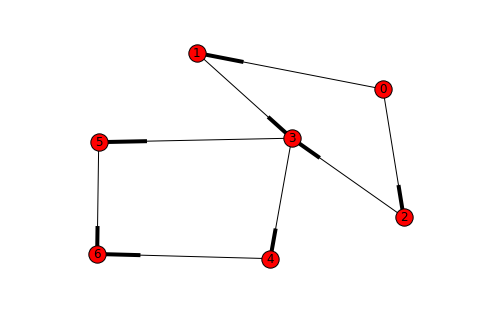Now lets do a depth first search (DFS) starting from node 0. There are several possible return values depending on which particular DFS function you call from the available ones. You can create a generator that returns the edges as the DFS

In :
T = nx.depth_first_search.dfs_tree(G, 0)

plt.figure()
nx.draw(T, with_labels=True)
plt.show()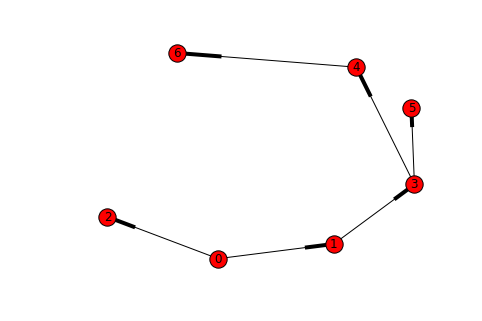In :
T = nx.depth_first_search.dfs_tree(G, 1)

plt.figure()
plt.subplot(121)
nx.draw(T, with_labels=True)
plt.subplot(122)
nx.draw(G, with_labels=True)
plt.show()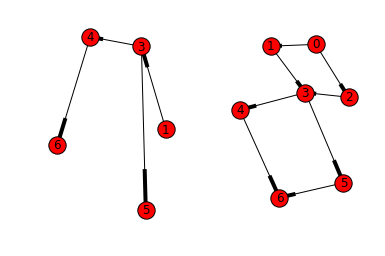By changing one line of code, we can also employ a different search method:

In :
T = nx.breadth_first_search.bfs_tree(G, 0)

plt.figure()
plt.subplot(121)
nx.draw(T, with_labels=True)
plt.subplot(122)
nx.draw(G, with_labels=True)
plt.show()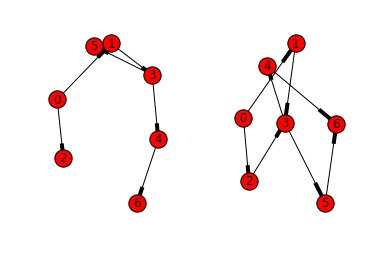### Shortest path¶

We'll model this problem on either an undirected or directed graph with weighted edges. Shortest path algorithms come in two flavors: single source and all pairs shortest path.

Single source shortest path computes the shortest paths to all other nodes from a particular starting node. For example, compute the best route from a starting location to and ending location is typically done this way. The classic algorithm for this is Dijkstra's algorithm. This problem is an example in which "greedy" algorithms don't work. That is, just repeatedly choosing the shortest edge you can see currently is not the right approach.

All pairs shortest path is one in which we want to compute the shortest path between any two pairs of nodes on the graph. This is typically done with the Floyd-Warshall algorithm.

We will use a weighted graph example from the Dijkstra's algorithm Wikipedia page.

In :
G = nx.Graph()

G.add_nodes_from([1, 2, 3, 4, 5, 6])
(1, 2, 7),
(2, 4, 15),
(4, 5, 6),
(5, 6, 9),
(6, 1, 14),
(1, 3, 9),
(2, 3, 10),
(3, 4, 11),
(3, 6, 2),
], weight='distance') # call the weight values 'distance'


Now we've defined the graph. In defining the edges we added a third argument which we are calling the 'distance'. We'll see how that's used now:

In :
S = nx.shortest_path_length(G, 1, weight='distance')
S

Out:
{1: 0, 2: 7, 3: 9, 4: 20, 5: 20, 6: 11}

This computes the distance between the starting node 1 and all the other nodes in the graph. If you wanted to get the actual paths rather than just the distances, you can use:

In :
P = nx.shortest_path(G, 2)
P

Out:
{1: [2, 1], 2: , 3: [2, 3], 4: [2, 4], 5: [2, 4, 5], 6: [2, 1, 6]}

This says that the shortest path from 1 to 5 goes through 1 -> 2 -> 4 -> 5.

### Minimal Spanning Tree¶

One of our motivating examples regarded an internet service provider who was laying down fiber optic cable to expand its services. We'll look a litte more carefully at how they may solve this problem.

Let's construct a simple "crossed-box" graph with weighted edges and try to compute a spanning tree of minimum weight in order to connect the network.

First we'll suppose that the weights come purely from the geometry of the box.One of our motivating examples regarded an internet service provider who was laying down fiber optic cable to expand its services. We'll look a litte more carefully at how they may solve this problem.

Let's construct a simple complete graph with 4 nodes whose geometry we will take to be that of a square. This graph will have weighted edges and we will try to compute a spanning tree of minimum weight in order to connect the network.

First we'll suppose that the weights come purely from the geometry of the graph in which the nodes are at the vertices of a square.

In :
G = nx.Graph()

(0, 1, 1.0),
(1, 2, 1.0),
(2, 3, 1.0),
(3, 0, 1.0),
(0, 2, 2.0**.5),
(1, 3, 2.0**.5),
])

T = nx.minimum_spanning_tree(G)

plt.figure()
nx.draw(G, with_labels=True)
plt.show()

plt.figure()
nx.draw(T, with_labels=True)
plt.show()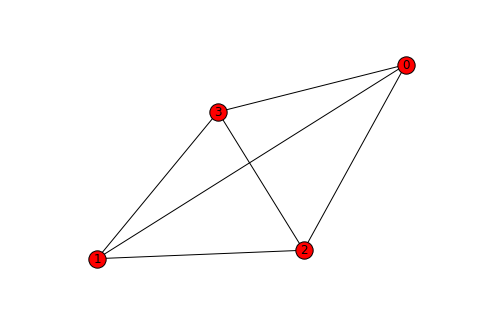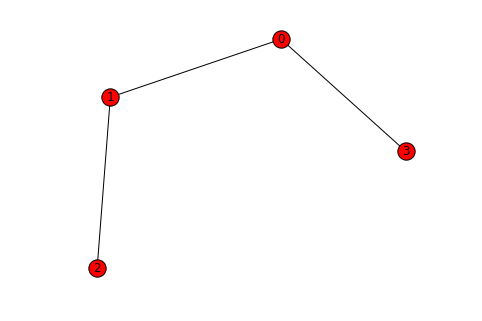Now, we suppose that there was already some infrastructure we could use to run cable from node 0 to 2. Let's see how that affects our solution.

In :
G.edge['weight'] = 0.4    # update the weight to take into account
# availible infrastructure

T = nx.minimum_spanning_tree(G) # recompute minimal spanning tree

plt.figure()
nx.draw(T, with_labels=True)
plt.show()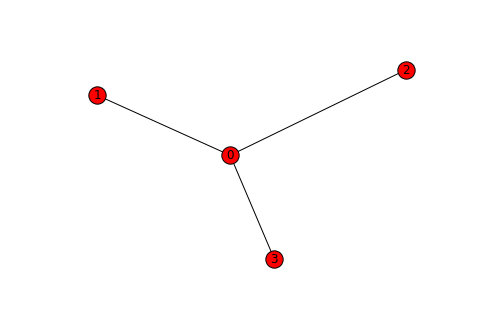### Max Flow / Min Cut¶

This time, we'll change terminology a little bit. We'll consider a graph which has certain capacities on its edges. For example, a road network may only be able to support a maximum amount of traffic. Or, a computer network may have limited bandwidth.

The goal is, we have two specials nodes called the source and the sink and we want to compute the maximum amount of "stuff" we can transport from the source to the sink. The constraint is, we cannot exceed the capacities of the edges and any node we use along the way must have the same amount of incoming "stuff" and outgoing "stuff".

If you'd like a more formal reference, take a look at this.

Let's try this on the simplest example: Just a single path.

In :
G = nx.DiGraph()

(0, 1, 4),
(1, 2, 3),
(2, 3, 6),

], weight='capacity')

plt.figure()
nx.draw(G, with_labels=True)
plt.show()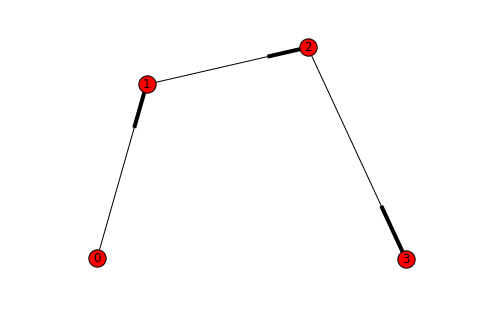On this graph, it's easy to see that the bottleneck is the edge 1 -> 2 which has capacity 3. Let's check this:

In :
nx.maximum_flow_value(G, 0, 3)

Out:
3

This algorithm is quite broad in its application. We'll see one easy example in today's projects.